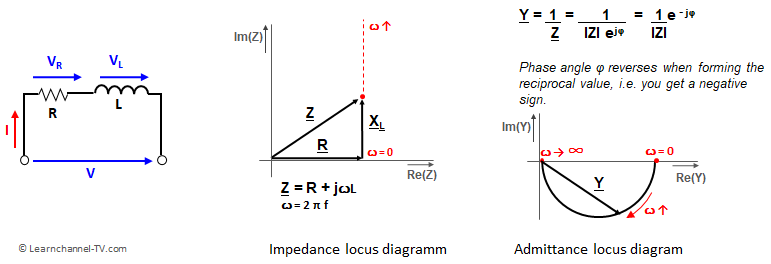# Locus Diagram in AC circuits

## Locus Diagram of RL Series Circuit

Locus diagrams show graphically the frequency dependence of admittances and impedances.

Locus diagram of  series R-L circuits

At f = 0 Hz, the inductive reactance according to XbL = j ꙍL has the value 0 Ω - thus the imaginary part of the impedance is zero. If the frequency increases, the inductive reactance also increases. → the pointer tip of the impedance moves on a straight line parallel to the imaginary axis.Locus diagram of series R-L circuits - impedance

You already recognize one disadvantage of the Locus Diagram of a RL Series Circuit: For big frequencies, i.e. ꙍ = 2πf, the reactive component becomes very large and thus the straight line of the locus curve also. A solution would be the representation of the admittance, i.e. the reciprocal of the impedance:Locus Diagram for Impedance and Admittance of a RL series circuit

For f = 0 Hz, only the ohmic resistance is effective in an RL-series circuit. As the frequency increases, the inductive reactance increases and the magnitude of the admittance Y decreases. The phase thereby moves from 0° to -90° with the pointer tip for Y moving on a semicircle.

1.•